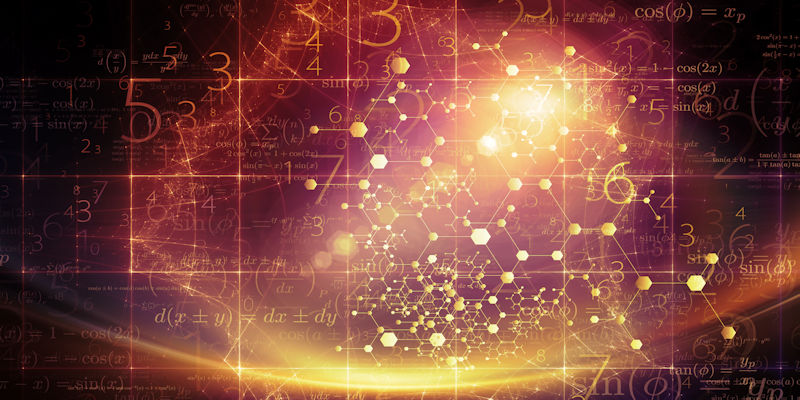# Statistical methodology and probabilityOur work on statistical methods develops new ideas for statistical estimation, inference and quantification of uncertainty. With the increasing importance of modern data science and “big data” problems, this research area studies the difficult theoretical problems underlying this work. This often requires analysis of the underlying probabilistic models, including models with spatial or network structure and in high dimensions.

### Our research interests

##### Statistics of shape and space:

“Shape analysis” describes our work developing methods to describe shapes and to summarise succinctly variability and changes through time. Applications include automatic chromosome identification and analysis, facial recognition in plastic surgery, fitting the outline of a human hand in a noisy image, and the description of growth in mouse vertebrae. These projects involve the development of suitable mathematical models including complex probability distributions, the use of deformable templates and analyses based on Kendall and Bookstein coordinates. Our work on spatial statistics is fundamental to a number of techniques used in image processing, for example, for locating gold/ore deposits, or creating maps. There are many unresolved theoretical problems in this area such as the behaviour of estimators and predictors, and development of better diagnostic tools.

##### High-dimensional statistics:

As encountered in many “big data” problems, often requires new and computational difficult techniques. Applications include search problems, image analysis and medical tomography. We are often in the so-called “p > n” regime where models have more parameters of interest than there are number of datapoints available. In these cases, we study “regularity” assumptions such as sparsity and dimension-reduction techniques, to produce accurate models for this data. We are interested in practical algorithms which are robust to noise in the data and are computationally efficient in very high dimensions, including the use of wavelets, functional data analysis, and random forest models.

##### Bayesian statistics:

Bayesian statistics provides a mathematical framework for understanding uncertainties in the real world. The idea of Bayesian statistics is to use observed data to update prior knowledge. This simple idea leads to deep philosophical and mathematical questions that have been the focus of much research attention over the past twenty-five years due to increased computing power and more important applications. Topics studied by our staff include: Bayesian networks, development of efficient posterior sampling, nonparametric regression techniques, and the quantification of expert analyses.

##### Networks:

Networks are an increasingly important area of probability and statistics. We study foundational issues relating to real-world networks, with our methods motivated by problems such as computer networks, wireless communications networks, and queueing networks. Networks are also an important conceptual tool to model relationships between objects, such as the Web graph of hyperlinks between webpages, and network models in biology.

##### Decision making:

We study tools for decision making and modelling of choices, including behaviour of agents, rational and irrational behaviour, and quantifying influence and expert analyses. We use probabilistic graphical models, Bayesian techniques, and game theory.

##### Probability:

Our research in probability involves the study of random structures, such as random graphs, random forests and random matrices. Study of these random combinatorial structures are motivated by foundational problems in networks, evolutionary biology, and chains of events. We also study extreme value modelling, which can help with the prediction of very rare “black swan” events.

### Further information

All upcoming seminars can be found on our events section.

### PhD projects

We have opportunities for prospective postgraduate researchers. Find out more.